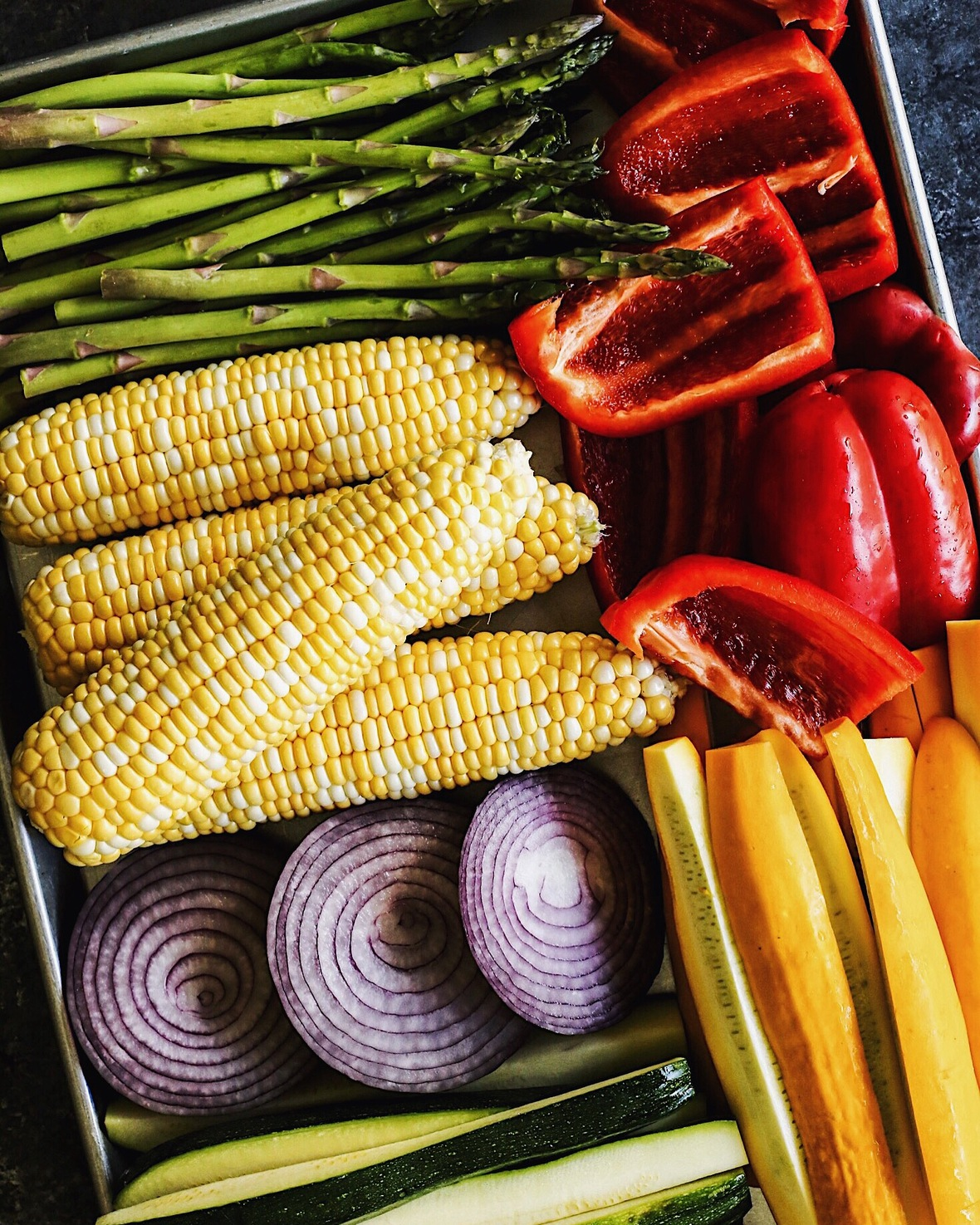table.module-1{width:75.47%;padding:0}table div table+table div table{width:75.47%;float:none;margin-left:auto;margin-right:auto;padding:0}table div table+table div table a{border:0 none;text-decoration:none}table div table+table div table img{width:100%!important;border:0 none;text-decoration:none}table div table+table div table td{width:100%;padding:0}/* styles */Follow along on Instagram and my Instagram story!

Here's The Latest #128
July 28, 2018

Hello! Welcome to the weekend!

It's been my idea of perfection weather-wise here in Minnesota lately, with temps in the upper 70's and lower 80's. Windows are open, people are in awesome good moods. Everyone wants to be outside!

Thank you for the emails last week, responding to my quest for the perfect chocolate chip cookie recipe. And for your interest in seeing us put together a post on that grilled beer can chicken we made last weekend - we are working on the recipe and hope to share it soon!

My Favorite Things this week... We grill nearly daily from early spring through late fall, and we have come to rely on these large and small rimmed pans (links HERE) for transporting food back and forth to/from the grill in the back yard. They are heavy and super durable, we don't have to worry about breaking them, and they wash up easy. Heck, we often serve food from the grill - directly from these pans - on our table. Why not?!

Thank you so much for joining us here each week, for reading the blog, and for following along on social media. We are grateful for your company.

Wishing you an awesome weekend!
Brenda

 table div table+table+table+table div table{width:100%;padding:0}table div table+table+table+table div table img{width:96.23%;padding:0;float:none}table div table+table+table+table div table td{width:100%;padding:0 1.88% 18px}/* styles */## NEW: On The Blog This Week

 table div table+table+table+table+table+table div table,table.module-5{width:65.09%;float:left;padding:0}table div table+table+table+table+table+table div table a{border:0 none;text-decoration:none}table div table+table+table+table+table+table div table img{width:100%!important;border:0 none;text-decoration:none}table div table+table+table+table+table+table div table td{width:100%;padding:0 20px 20px 0}/* styles */ I'm calling this our Salad of Summer 2018! This Grilled Vegetable Salad features veggies charred to perfection and then drizzled with a bright-and-sunny herby lemon vinaigrette. Bites of creamy, salty blue cheese (or substitute Parmesan) are the finishing touch! Read this post and grab the recipe here.
 table div table+table+table+table+table+table+table div table{width:100%;padding:0}table div table+table+table+table+table+table+table div table img{width:96.23%;padding:0;float:none}table div table+table+table+table+table+table+table div table td{width:100%;padding:0 1.88% 18px}/* styles */## CRUMBS: What We Made Recently

 table div table+table+table+table+table+table+table+table+table div table,table.module-8{width:65.09%;float:left;padding:0}table div table+table+table+table+table+table+table+table+table div table a{border:0 none;text-decoration:none}table div table+table+table+table+table+table+table+table+table div table img{width:100%!important;border:0 none;text-decoration:none}table div table+table+table+table+table+table+table+table+table div table td{width:100%;padding:0 20px 20px 0}/* styles */ We make this Chinese Chicken Salad every single summer...although there's absolutely no reason we shouldn't be making it year 'round. It's so good, a family favorite! Get the recipe here.
 table div table+table+table+table+table+table+table+table+table+table div table,table.module-9{width:65.09%;float:left;padding:0}table div table+table+table+table+table+table+table+table+table+table div table a{border:0 none;text-decoration:none}table div table+table+table+table+table+table+table+table+table+table div table img{width:100%!important;border:0 none;text-decoration:none}table div table+table+table+table+table+table+table+table+table+table div table td{width:100%;padding:0 20px 20px 0}/* styles */ Looking for a super easy way to make grilled pizzas? Grab a package of Flat Out pizza crusts and add your favorite toppings!
 table div table+table+table+table+table+table+table+table+table+table+table div table,table.module-10{width:65.09%;float:left;padding:0}table div table+table+table+table+table+table+table+table+table+table+table div table a{border:0 none;text-decoration:none}table div table+table+table+table+table+table+table+table+table+table+table div table img{width:100%!important;border:0 none;text-decoration:none}table div table+table+table+table+table+table+table+table+table+table+table div table td{width:100%;padding:0 20px 20px 0}/* styles */ I have bought (and eaten!) more watermelon this summer than any other. Every one I buy is so good! I made this Watermelon, Arugula, and Pickled Onion Salad this week - super yummy! The recipe is from Food 52. (The only thing I changed was to add a little bit of honey to the dressing.)
 table div table+table+table+table+table+table+table+table+table+table+table+table div table{width:100%;padding:0}table div table+table+table+table+table+table+table+table+table+table+table+table div table img{width:96.23%;padding:0;float:none}table div table+table+table+table+table+table+table+table+table+table+table+table div table td{width:100%;padding:0 1.88% 18px}/* styles */## ARCHIVES: Yum From The Past

 table div table+table+table+table+table+table+table+table+table+table+table+table+table+table div table,table.module-13{width:65.09%;float:left;padding:0}table div table+table+table+table+table+table+table+table+table+table+table+table+table+table div table a{border:0 none;text-decoration:none}table div table+table+table+table+table+table+table+table+table+table+table+table+table+table div table img{width:100%!important;border:0 none;text-decoration:none}table div table+table+table+table+table+table+table+table+table+table+table+table+table+table div table td{width:100%;padding:0 20px 20px 0}/* styles */ Sweet and smoky and beautifully spiced, this Grilled Chipotle-Orange Salmon promises to become a grilling season obsession! Get the recipe here.
 table div table+table+table+table+table+table+table+table+table+table+table+table+table+table+table div table,table.module-14{width:65.09%;float:left;padding:0}table div table+table+table+table+table+table+table+table+table+table+table+table+table+table+table div table a{border:0 none;text-decoration:none}table div table+table+table+table+table+table+table+table+table+table+table+table+table+table+table div table img{width:100%!important;border:0 none;text-decoration:none}table div table+table+table+table+table+table+table+table+table+table+table+table+table+table+table div table td{width:100%;padding:0 20px 20px 0}/* styles */ Fresh Raspberry and Brownie Chunk Ice Cream, with bright, sweet raspberries in every bite! Get the recipe here.
 table div table+table+table+table+table+table+table+table+table+table+table+table+table+table+table+table div table,table.module-15{width:65.09%;float:left;padding:0}table div table+table+table+table+table+table+table+table+table+table+table+table+table+table+table+table div table a{border:0 none;text-decoration:none}table div table+table+table+table+table+table+table+table+table+table+table+table+table+table+table+table div table img{width:100%!important;border:0 none;text-decoration:none}table div table+table+table+table+table+table+table+table+table+table+table+table+table+table+table+table div table td{width:100%;padding:0 20px 20px 0}/* styles */ A much easier twist on tacos al pastor, these Grilled Pork and Pineapple Tacos promise to be your next taco night hit! Get the recipe here.
 table div table+table+table+table+table+table+table+table+table+table+table+table+table+table+table+table+table div table,table.module-16{width:65.09%;float:left;padding:0}table div table+table+table+table+table+table+table+table+table+table+table+table+table+table+table+table+table div table a{border:0 none;text-decoration:none}table div table+table+table+table+table+table+table+table+table+table+table+table+table+table+table+table+table div table img{width:100%!important;border:0 none;text-decoration:none}table div table+table+table+table+table+table+table+table+table+table+table+table+table+table+table+table+table div table td{width:100%;padding:0 20px 20px 0}/* styles */ The recipe for these Prosecco Margaritas is in big-batch cocktail form, perfect for entertaining. And you just have to love the bubbles! Get the recipe here.
 table div table+table+table+table+table+table+table+table+table+table+table+table+table+table+table+table+table+table div table,table.module-17{width:64.91%;float:left;padding:0}table div table+table+table+table+table+table+table+table+table+table+table+table+table+table+table+table+table+table div table a{border:0 none;text-decoration:none}table div table+table+table+table+table+table+table+table+table+table+table+table+table+table+table+table+table+table div table img{width:100%!important;border:0 none;text-decoration:none}table div table+table+table+table+table+table+table+table+table+table+table+table+table+table+table+table+table+table div table td{width:100%;padding:0 20px 20px 0}/* styles */ These Extra-Marshmallow Rice Krispies Bars with Confetti are soft and gooey-chewy, with a tinted white chocolate glaze and fun mix of sprinkles! Get the recipe here.
 Like   Tweet   Pin   +1